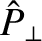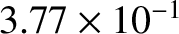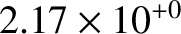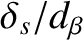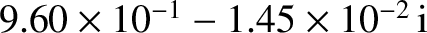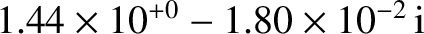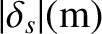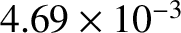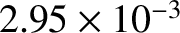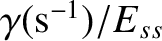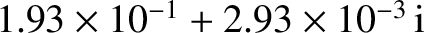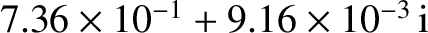# Resonant Layer Thickness

According to Equations (3.102) and (3.103), the dispersion relation of a magnetic perturbation interacting with a thin, rigid, resistive wall can be written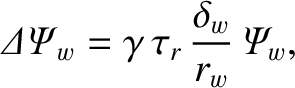(6.23)

where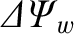is a measure of the current flowing in the wall,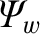the perturbed magnetic flux that penetrates the wall,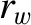the wall minor radius,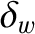the wall radial thickness, and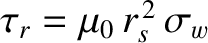the time required for magnetic flux to diffuse a distancethrough the wall material. Here,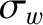is the electrical conductivity of the wall material. By analogy with the previous result, we would expect the dispersion relation of a magnetic perturbation interacting with the thin resistive layer that surrounds the rational surface to take the form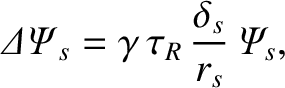(6.24)

where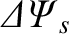is a measure of the current flowing in the layer,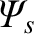the perturbed magnetic flux that penetrates the layer,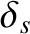is the radial thickness of the layer, and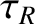is the time required for magnetic flux to diffuse a distance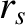through the plasma. [See Equation (5.49).] Note that, in general,is a complex quantity. In fact, the true layer thickness is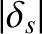. It follows from Equation (3.74) that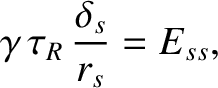(6.25)

where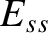is the real tearing stability index. Finally, Equations (5.48), (5.66), (6.2), and (6.4) can be combined with the previous equation to give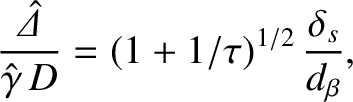(6.26)

where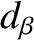is the ion sound radius at the rational surface. [See Equation (4.75).]

Table: 6.2 Normalized dimensionless layer parameters, layer thicknesses, and tearing mode growth-rates in a low-field and a high-field tokamak fusion reactor. See Equations (6.31)–(6.33). Here,is the complex layer thickness,the actual layer thickness,the ion sound radius,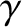the complex growth-rate in a frame of reference that co-rotates with the electron fluid at the rational surface, andthe tearing stability index.
 Low-Field High-Field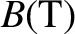5.0 12.0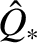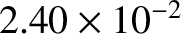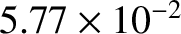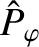1.15 6.62